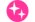IEICE Electronics Express
Online ISSN : 1349-2543
ISSN-L : 1349-2543
Acceleration of nonequilibrium Green's function simulation for nanoscale FETs by applying convolutional neural network model
Satofumi SoumaMatsuto Ogawa

ジャーナル フリー 早期公開

この記事には本公開記事があります。The final version of this article is now available: Vol. 17 (2020), No. 4 pp. 20190739-20190739

We investigate the application of convolutional neural networks (CNNs) to accelerate quantum mechanical transport simulations (based on the nonequilibrium Green's function (NEGF) method) of double-gate MOSFETS. In paricular, given a potential distribution as input data, we implement the convolutional autoencoder to train and predict the carrier density and local quantum capacitance distributions. The results indicate that the use of a single trained CNN model in the NEGF self-consistent calculation along with Poisson's equation produces accurate potentials for a wide range of the gate lengths, and all within a significantly shorter computational time than the conventional NEGF calculations.著者関連情報
© 2020 by The Institute of Electronics, Information and Communication Engineers
Top